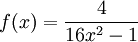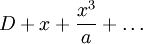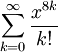# Quiz 3

Previous: Quiz 2

Next: Contact

The following quiz covers topics in Unit 3.

If you would like to know how well you did on the quiz, you can have the quiz results sent to an email address that you provide at the end of the quiz. You will also be sent partial solutions.

## Quiz 3: Power Series

Progress:

The following function can be represented as a power seriesWhat is the radius of convergence of this power series?

The second term in the Maclaurin expansion of

f(x) = e9xcos(5x)

is ax. What is the value of a?

It can be shown that the integralcan be written aswhere D is a constant. What is the value of a?

What is the radius of convergence of the Maclaurin series ofWhat is the sum of this infinite seriesPrevious: Quiz 2

Next: Contact